## Sigma 7 Pulse and Direction Drive

#### Wiring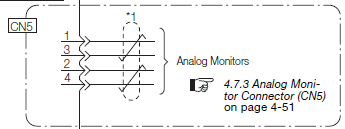Use a cable as shown below: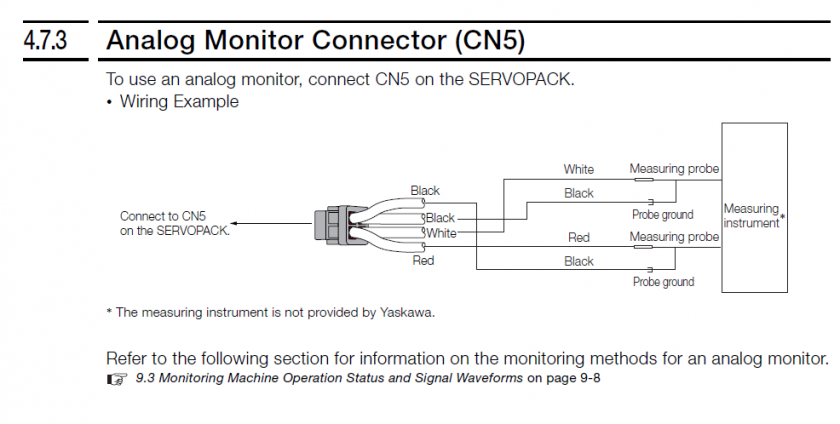#### Setup Options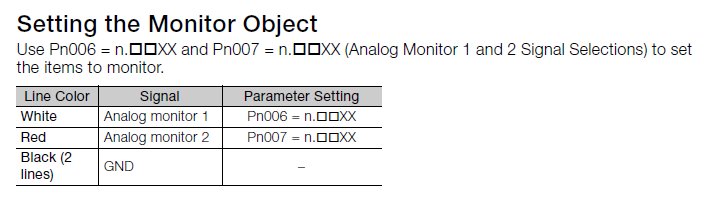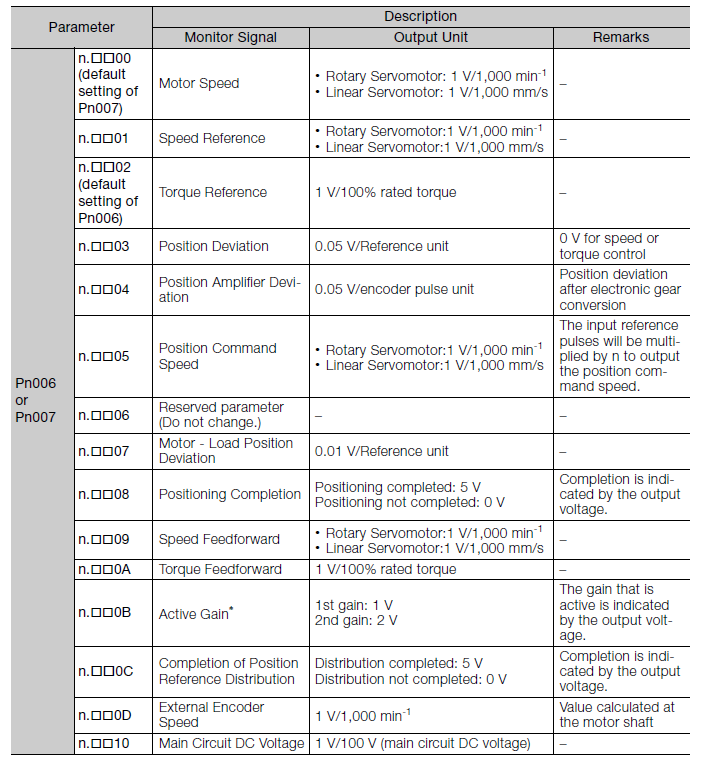Factor and Offset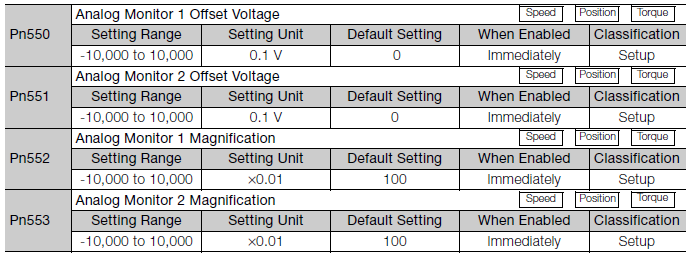Analog Monitor 1

Pn006=.XX02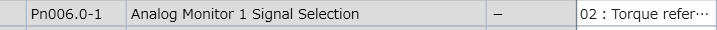Calculate the value of Pn552 based on the max rated torque you will ever see on this device:

Pn552 = 10V / (0.01 * Max Rated Torque %)

 Max Rated Torque % Pn552 40% 25.00 50% 20.00 100% 10.00 300% 03.33

For example, if you want to see up to 50% of rated torque, calculate it as follows:

Pn552 = 10V / (0.01 * 50%) = 20.00 so you would set Pn552 to 20.00.

Proof:

Actual Output Voltage = Pn552*0.01 * Actual Rated Torque %

Substitute Max Output Voltage for Actual Output Voltage and Max Torque for Actual Rated Torque:

Max Output Voltage = Pn552*0.01 * Max Rated Torque %

Solve for Pn552:

Pn552 = Max Output Voltage / (0.01 * Max Rated Torque %)

Set Max Output Voltage to 10V:

Pn552 = 10V / (0.01 * Max Rated Torque %)

#### Mach4 Setup

If using the Siemens PLC, see this document: Siemens PLC - Wiring SM1234.

Reference Manual: See attached Sigma-7 Manual Analog-Pulse.pdf